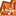# App Development

Announcements
Read about the latest Qlik Community enhancements on the Community News blog!
cancel
Showing results for
Did you mean:Creator

## Re: How can add "If and" condition in pivot table in Qliksense

4th formula

If (Client_type=Individual and country = 'A', (Sum(VALUES)-(value))/sum(VALUES),

If (Client_type=Individual and country = 'B', (Sum(VALUES)-(value))/sum(VALUES),

NULL()))

I used above formula in pivot table but I am getting negative values in % column, that is not correct. somebody help me to fix that issue ..I want to  fix this issue in the next 48 hours.

Awiting Solution on  this error..

Thanks in advance for quick response

19 RepliesMVP

Would you be able to share a sample to visualize your issue?Creator
Author

Yes..I am using excel dataMVP

That is great, would you be able to share the Excel and if possible also share the expected output?Creator
Author

I have used IF OR condition but I am getting only  % of country and other country showing wrong calculation.

do you want sample data excelsheetMVP

Something to make sense of your question....Creator
Author

where is option for attached?MVP

Check here:Creator
Author

Please find the attached excel sheet. Sheet A and B containing month wise dataTotal_a and Total_b containg total count of data..This is sample template of random data.

Formula for calculation is using as

(Total Value - Value for DE) / Total ValueCreator
Author

I have attached the data..

I am using below script

Table_a:

crosstable(Month,Value,5)

DE,
"FCC 20",
CATEEGORY,
"DQ DIM",
Country,

"42711",

"42742",

"42773",

"42801",

"42832",

"42862",

"42893",

"42923",

"42954",

"42985",

"43015",

"43046",

"43076"

FROM [lib://HK/HK_Sales_1.xlsx]
(ooxml, embedded labels, table is Sheet1);

Total_a:

crosstable(Month,Values,1)

"Total",

"42711",

"42742",

"42773",

"42801",

"42832",

"42862",

"42893",

"42923",

"42954",

"42985",

"43015",

"43046",

"43076"

FROM [lib://HK/HK_Sales_1.xlsx]

(ooxml, embedded labels, table is a);

Table_b:

crosstable(Month,Value,5)

DE,
"FCC 20",
CATEEGORY,
"DQ DIM",
Country,

"42711",

"42742",

"42773",

"42801",

"42832",

"42862",

"42893",

"42923",

"42954",

"42985",

"43015",

"43046",

"43076"

FROM [lib://HK/HK_Sales_1.xlsx]
(ooxml, embedded labels, table is Sheet1);

Total_b:

crosstable(Month,Values,1)  -------------- Change the name as BValues instead of Values

"Total",

"42711",

"42742",

"42773",

"42801",

"42832",

"42862",

"42893",

"42923",

"42954",

"42985",

"43015",

"43046",

"43076"

FROM [lib://HK/HK_Sales_1.xlsx]

(ooxml, embedded labels, table is a);

I am using three tables currently. But visualization part not giving  correct result for calculation in the pivot table.

by using If condition:

If (Client_type=Individual and country = 'A', (Sum(VALUES)-(value))/sum(VALUES),

If (Client_type=Individual and country = 'B', (Sum(VALUES)-(value))/sum(VALUES),

NULL()))

Note: I have change the name of Values in the 2nd table as BValuesTags
Community Browser